Testing of a RLC Neon Transformer Protection Network for Tesla Coil Primary Circuit Applications

Terry Fritz

3-3-98

Summary:

When used in Tesla coil primary circuits, neon sign transformers often fail due to high-frequency voltage and current stresses. In order to protect the transformer from these stresses, various filter networks can be used to block destructive signals from reaching the transformers secondary windings. This paper describes testing that was done using a simple RLC filter network. Voltage and current waveforms measured across several of the components are presented. This filter design exhibited serious problems when tested.

Experiment:

This experiment measures the voltages and current waveforms developed across a 15000 volt, 60 mA neon sign transformer as it is driving a Tesla coil primary circuit through a LRC filter network. The network has a 940pF capacitor across the transformer’s output. There is a 1000 ohm resistor in one leg and a 26.7uH inductor in the other leg going to the primary circuit. The output is connected across the primary capacitor since previous testing has shown this to be a worst case connection. There is no secondary inductor in place for this experiment. The output voltage of the transformer is limited to about 3000VRMS to protect the equipment. The voltage waveform across the filter inductor and primary capacitor is also measured for reference.

Setup:

The experimental setup is shown in the block diagram below. The primary capacitor is a 17.25nF 30KV ceramic capacitor bank. The primary inductor is a 120uH linear coil inductor. The spark gap is a simple 0.02 inch gap that has holes drilled so that air can be pumped into the gap. The transformer is a standard 15KV 60mA neon sign transformer.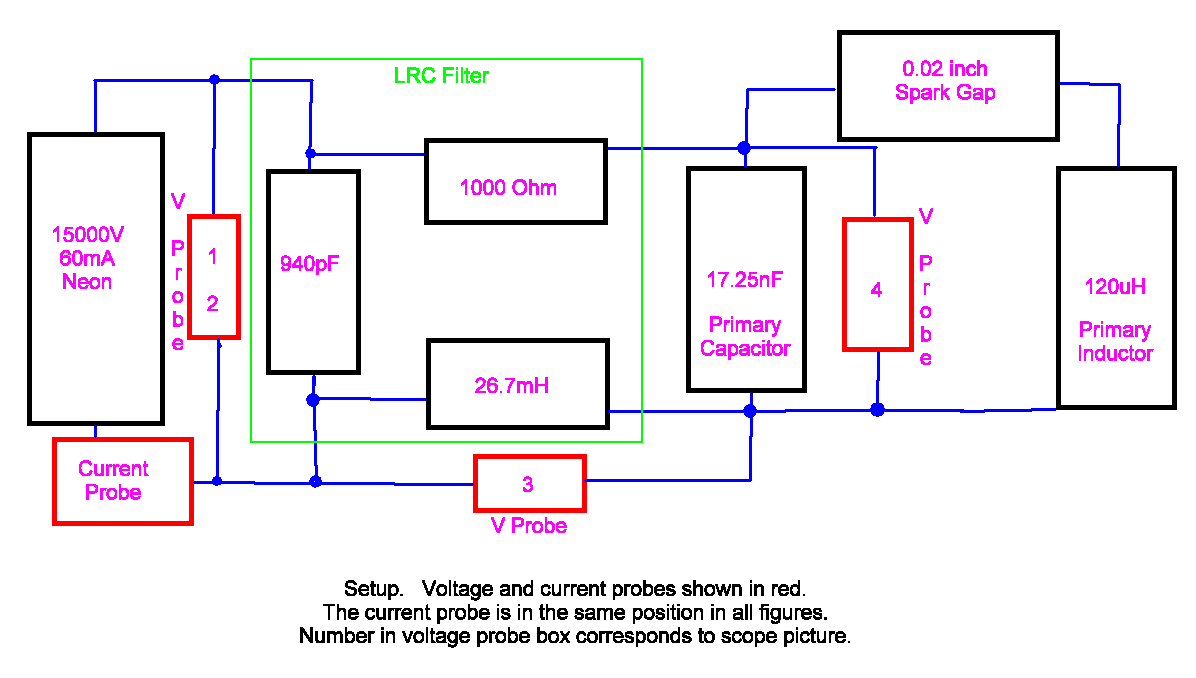Block Diagram of Test Setup

Construction:

The filter consists of three parts. There is a single capacitor that is placed across the output of the transformer. This capacitor is a 940pF, 30KV doorknob style ceramic capacitor. There is also one 1000 ohm 250 watt power resistor and one 26.7mH ferrite inductor placed between the 940pF capacitor and the primary circuitry.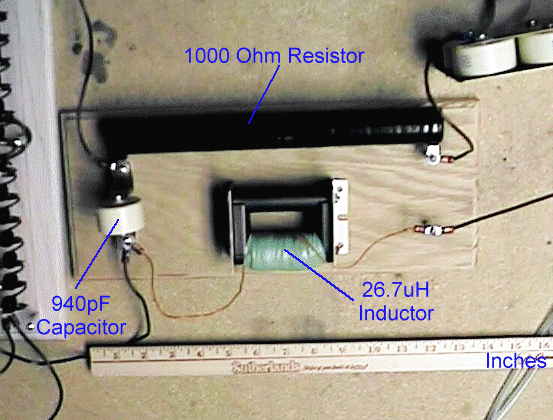Picture of Filter Circuit

The inductor consists of 92 turns of #22 solid copper wire wound on a 3 x 3.5 inch ferrite core. The inductance measures 26.7uH. Each layer of winding is cover with PVC electrical tape to provide a degree of high voltage insulation.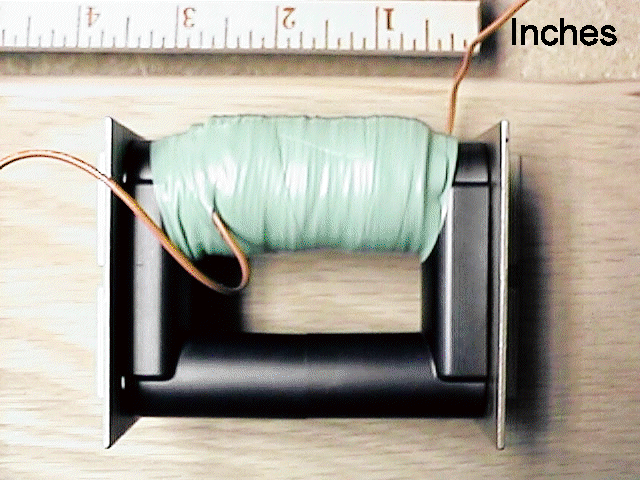Ferrite Inductor

Results:

The maximum voltages used in this testing were limited to about 3000 VRMS to prevent damage to the transformer.

The first set of pictures (Figures 1 and 2) show the voltage and current waveforms of the transformer’s output with the filter network in place.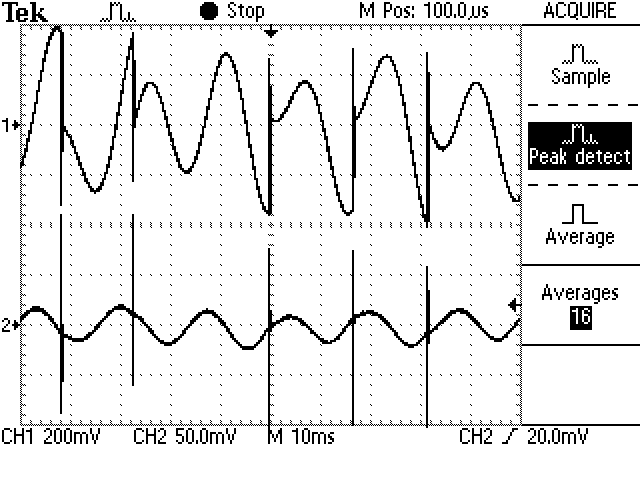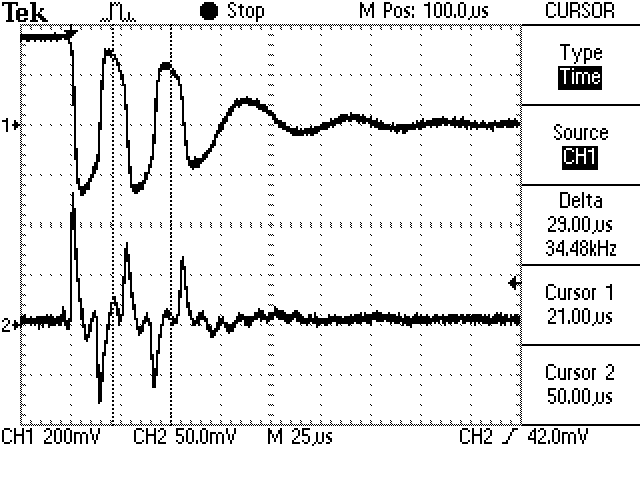Figures 1 and 2. Voltage and current waveforms across the transformer.

Top Trace Voltage 2KV/div

Bottom Trace Current 50mA/div

Note that the voltage across the transformer shows an ~3KV, ~34KHz oscillation. The resonant frequency of the filter inductor and capacitor is approximately this frequency:

F = 1 / (2*pi*sqrt(L*C)) == 1 / (2*pi*sqrt(26.7e-3*940e-12)) == 31.76KHz

The 110KHz primary frequency appears to be filtered out rather well. However, there is a heavy 130mA initial current spike and substantial following current spikes on the transformer output. These also have a frequency of approximately 34KHz.

Figure 3 shows the same situation with voltage probe across the 26.7uH inductor.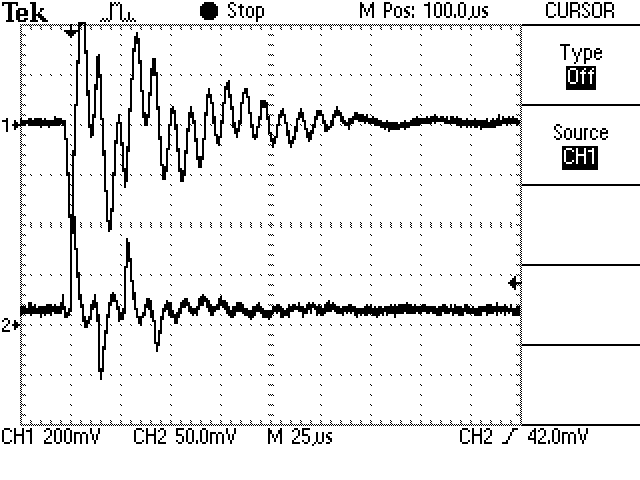Figure 3. Voltage waveform across the 26.7uH inductor. The current is still measured at the transformer’s output.

Top Trace Voltage 2KV/div

Bottom Trace Current 50mA/div

Figure 4 shows the same situation with voltage probe across the 17.25nF primary capacitor.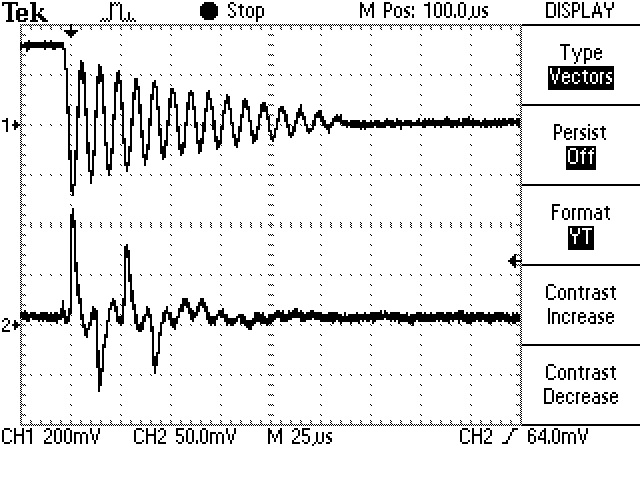Figure 4. Voltage waveform across the primary (17.25nF) capacitor. The current is still measured at the transformer’s output.

Top Trace Voltage 2KV/div

Bottom Trace Current 50mA/div

Conclusion:

The filter network effectively reduced the 110KHZ high frequency voltages and currents on the transformers secondary windings. However, the network appears to have created a similar 34KHz signal in it’s place. This signal is as potentially damaging as the original signal.